Courses

# Test: Analog Electronics Circuits

## 25 Questions MCQ Test Basic Electronics Engineering for SSC JE (Technical) | Test: Analog Electronics Circuits

Description
This mock test of Test: Analog Electronics Circuits for Electrical Engineering (EE) helps you for every Electrical Engineering (EE) entrance exam. This contains 25 Multiple Choice Questions for Electrical Engineering (EE) Test: Analog Electronics Circuits (mcq) to study with solutions a complete question bank. The solved questions answers in this Test: Analog Electronics Circuits quiz give you a good mix of easy questions and tough questions. Electrical Engineering (EE) students definitely take this Test: Analog Electronics Circuits exercise for a better result in the exam. You can find other Test: Analog Electronics Circuits extra questions, long questions & short questions for Electrical Engineering (EE) on EduRev as well by searching above.
QUESTION: 1

Solution:
QUESTION: 2

Solution:
QUESTION: 3

### To prevent a DC return between source and load, it is necessary to use

Solution:
QUESTION: 4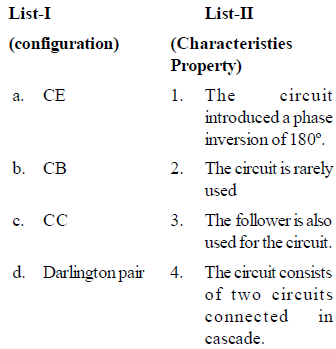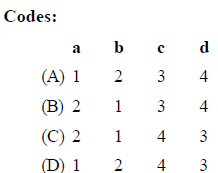Solution:

Explanation : a) The common emitter amplifier is INVERTING. The inversion is equivalent to 180 degree phase shift. The inversion can be easily seen intuitively without any complex circuit analysis.

b) CB will not provide current gain and input impedance is very low, output impedance is very high therefore it is not used in many applications.

c) The common collector or grounded collector configuration is commonly used where a high impedance input source needs to be connected to a low impedance output load requiring a high current gain.

d) The Darlington transistor is a special type of transistor with a high current gain. It consists of two internally bipolar transistors being connected in cascade.

QUESTION: 5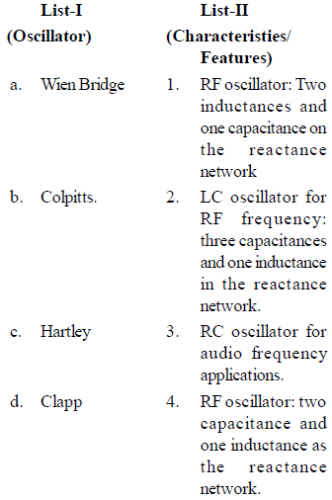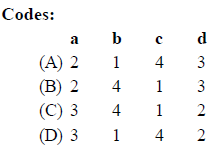Solution:
QUESTION: 6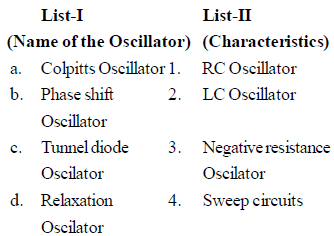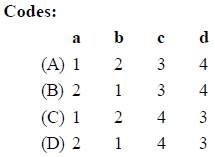Solution:
QUESTION: 7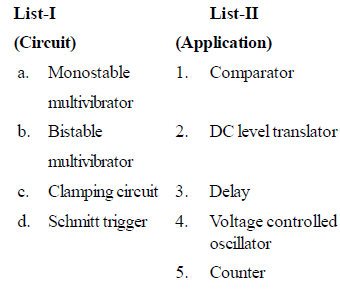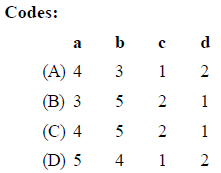Solution:
QUESTION: 8

Thermal runaway will take place if the quiescent point is such that

Solution:
QUESTION: 9

The given circuit has a feedback factor of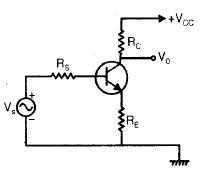Solution:
QUESTION: 10

CE configuration is the most preferred transistor configuration when used as a switch because it

Solution:
QUESTION: 11

Consider the following statements regarding a common emitter amplifier. It can be converted into an oscillator by:

2. phase shifting the output by 180° and feeding this phase-shifted output to the input

3. using only a series tuned circuit as a load on the amplifier

4. using a negative resistance device as a load on the amplifier

Which of the above statements are correct?

Solution:
QUESTION: 12

The PIV rating of the diodes used in power supply circuits are chosen by which one of the following criteria? (Vm is the peak input supply voltage to the rectifier circuit used in the power supply)

Solution:
QUESTION: 13

An RC amplifier stage has a bandwidth of 500  kHz. What will be the rise time of this amplifier stage?

Solution:
QUESTION: 14

Consider the following statements in respect of a transistor R-C coupled amplifier:

1. The low frequency response is determined by the transistor junction capacitors.

2. The high frequency response is limited by coupling capacitors.

3. The Miller capacitance reduces the gain at high frequencies.

4. As the gain is increased the bandwidth gets reduced.

Which of these statements are correct?

Solution:
QUESTION: 15

A CE-amplifier has RL = 10 kΩ. Given hie = 1 kΩ, hfe = 50, hre = 0 and 1/hoe = 40 kΩ. What is the voltage gain?

Solution:
QUESTION: 16

In the given circuit, D1 is an ideal germanium diode and D2 is a silicon diode having its cut-in voltage as 0.7 V, forward resistance as 20Ω and reverse saturation current (ls) as 10 nA. What are the values of I and V for this circuit, respectively?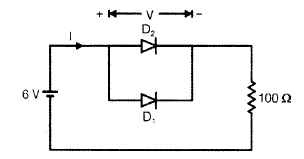Solution:
QUESTION: 17

A tuned amplifier has peak output at 2 MHz and quality factor 50. The bandwidth and 3-dB frequencies shall be at what values respectively?

Solution:
QUESTION: 18

Consider the following statements: Tuned amplifiers

1. are wide band amplifiers

3. performance is determined by Q of the circuit

Which of the statements given above are correct?

Solution:
QUESTION: 19

A feed back amplifier has an open loop gain of - 100. If 4% of the output is fed back in a degenerative loop, what is the closed loop gain of the amplifier?

Solution:
QUESTION: 20

Which one of the following statements is not correct with regard to power amplifiers ?

Solution:
QUESTION: 21

Consider the following statements regarding the class-B power amplifiers (Complementarysymmetry type):

1. The efficiency of the amplifier is higher than that of class-A amplifier

.2. The power output is low.

3. Cross over distortion is present

.4. The standby power dissipation is absent.

Which of the above statements are correct?

Solution:
QUESTION: 22

Consider the following statements :The bias stability of an emitter-bias amplifier circuit improves by

1. decreasing the value of RB.

2. increasing the value of RE.

3. decreasing the value of Re.

4. increasing the value of Rb.

5. Increasing the value of Rc.

Which of the above statements are correct ?

Solution:
QUESTION: 23

Consider the following statements:

When compared with a bridge rectifier, a centre tapped full wave rectifier:

1. Has larger transformer utilization factor.

2. Can be used for floating output terminalsi.e. no input terminal is grounded.

3. Needs two diodes instead of four.

4. Needs diodes of a lower PIV rating.

Which of these statements is/are correct?

Solution:
QUESTION: 24

The output Vdc from the below circuits is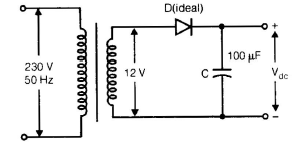Solution:
QUESTION: 25

A small signal voltage amplifier in common emitter configuration was working satisfactorily.Suddenly its emitter-bypass capacitor (CE) got disconnected. Its:

1. Voltage gain will decrease

2. Voltage gain will increase

3. Bandwidth will decreases

4. Bandwidth will increase

Which of these statements are correct?

Solution: